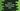# Python calendar module HTMLCalendar class## Introduction :

Python calendar module provides one HTMLCalendar class to get one HTML calendar. In this post, I will show you how to use the HTMLCalendar class with an example. It also provides different methods to get different types of HTML calendar results. This method is really useful if you want to get one calendar as a HTML.

### Syntax of calendar.HTMLCalendar :

Following is the syntax of HTMLCalendar :

``calendar.HTMLCalendar(firstWeekDay)``

It returns one instance of HTMLCalendar class. We can use this instance to get one month or year’s calendar as HTML result. Following are the methods defined in this class :

### formatmonth(year, month, withyear=True) :

This method is used to get one month’s calendar as HTML. Here, year is the year of the calendar, month is the month of the calendar and withyear is a boolean that defines if we need the year to include in the HTML header or not. If we mark it as False, it will return only the month in the header.

Let’s consider the below example :

``````import calendar

cal = calendar.HTMLCalendar(0)

html_result = cal.formatmonth(2020, 1, True)

print(html_result)``````

The output htmlresult_ will give one output like below :

### formatyear(year,width=3) :

This method returns one html calendar for the provided year. The second parameter width is the number of months we want to show in the calendar. The default value is 3. Let’s consider the below example :

``````import calendar

cal = calendar.HTMLCalendar(0)

html_result = cal.formatyear(2020, 4)

print(html_result)``````

It will give the below output :

### formatyearpage(year, width=3, css=‘calendar.css’, encoding=None) :

This method returns one full HTML page. year is the year for the html, width is the number of rows we are showing in the HTML, css is the css file we are using using for the output HTML and encoding is used to define the encoding of the output HTML file.

None can be used to the css field if you don’t want to use any css and encoding can be pass as None to use the default encoding. By default, encoding is None.